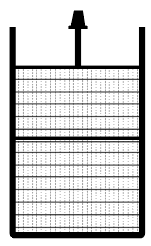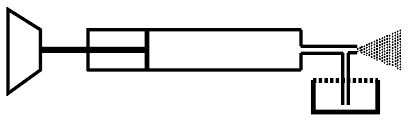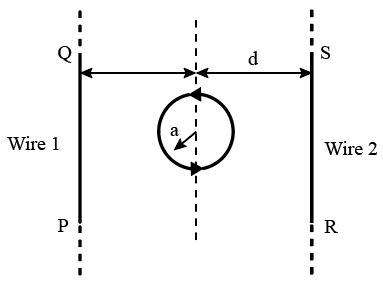Instructions

In the figure a container is shown to have a movable (without friction) piston on top. The container and the piston are all made of perfectly insulating material allowing no heat transfer between outside and inside the container. The container is divided into two compartments by a rigid partition made of a thermally conducting material that allows slow transfer of heat. The lower compartment of the container is filled with 2 moles of an ideal monatomic gas at 700 K
and the upper compartment is filled with 2 moles of an ideal diatomic gas at 400 K. The heat capacities per mole of an ideal monatomic gas are $$C_v = \frac{3}{2}R, C_P = \frac{5}{2}R,$$ and those for an ideal diatomic gas are $$C_v = \frac{5}{2}R, C_P = \frac{7}{2}R.$$Question 11

Question 12

# Now consider the partition to be free to move without friction so that the pressure of gases in both compartments is the same. Then total work done by the gases till the time they achieve equilibrium will be

Instructions

A spray gun is shown in the figure where a piston pushes air out of a nozzle. A thin tube of uniform cross section is connected to the nozzle. The other end of the tube is in a small liquid container. As the piston pushes air through the nozzle, the liquid from the container rises into the nozzle and is sprayed out. For the spray gun shown, the radii of the piston and the nozzle are 20 mm and 1mm, respectively. The upper end of the container is open to the atmosphere.Question 13

Question 14

# If the density of air is $$\rho_a$$ and that of the liquid $$\rho_l$$, then for a given piston speed the rate (volume per unit time) at which the liquid is sprayed will be proportional to

Instructions

The figure shows a circular loop of radius a with two long parallel wires (numbered 1 and 2) all in the plane of the paper. The distance of each wire from the centre of the loop is d. The loop and the wires are carrying the same current I. The current in the loop is in the counterclockwise direction if seen from above.Question 15

Question 16

# Consider $$d \gg a$$, and the loop is rotated about its diameter parallel to the wires by $$30^\circ$$ from the position shown in the figure. If the currents in the wires are in the opposite directions, the torque on the loop at its new position will be (assume that the net field due to the wires is constant over the loop)

Instructions

For the following questions answer them individually

Question 17

Question 18

Question 19

Question 20

OR